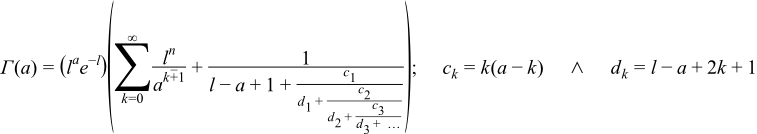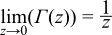#Boost C++ Libraries

...one of the most highly regarded and expertly designed C++ library projects in the world.

### Gamma

##### Synopsis
```#include <boost/math/special_functions/gamma.hpp>
```
```namespace boost{ namespace math{

template <class T>
calculated-result-type tgamma(T z);

template <class T, class Policy>
calculated-result-type tgamma(T z, const Policy&);

template <class T>
calculated-result-type tgamma1pm1(T dz);

template <class T, class Policy>
calculated-result-type tgamma1pm1(T dz, const Policy&);

}} // namespaces
```
##### Description
```template <class T>
calculated-result-type tgamma(T z);

template <class T, class Policy>
calculated-result-type tgamma(T z, const Policy&);
```

Returns the "true gamma" (hence name tgamma) of value z:The final Policy argument is optional and can be used to control the behaviour of the function: how it handles errors, what level of precision to use etc. Refer to the policy documentation for more details.

There are effectively two versions of the tgamma function internally: a fully generic version that is slow, but reasonably accurate, and a much more efficient approximation that is used where the number of digits in the significand of T correspond to a certain Lanczos approximation. In practice any built in floating point type you will encounter has an appropriate Lanczos approximation defined for it. It is also possible, given enough machine time, to generate further Lanczos approximation's using the program libs/math/tools/lanczos_generator.cpp.

The return type of this function is computed using the result type calculation rules: the result is `double` when T is an integer type, and T otherwise.

```template <class T>
calculated-result-type tgamma1pm1(T dz);

template <class T, class Policy>
calculated-result-type tgamma1pm1(T dz, const Policy&);
```

Returns `tgamma(dz + 1) - 1`. Internally the implementation does not make use of the addition and subtraction implied by the definition, leading to accurate results even for very small `dz`. However, the implementation is capped to either 35 digit accuracy, or to the precision of the Lanczos approximation associated with type T, whichever is more accurate.

The return type of this function is computed using the result type calculation rules: the result is `double` when T is an integer type, and T otherwise.

The final Policy argument is optional and can be used to control the behaviour of the function: how it handles errors, what level of precision to use etc. Refer to the policy documentation for more details.

##### Accuracy

The following table shows the peak errors (in units of epsilon) found on various platforms with various floating point types, along with comparisons to the GSL-1.9, GNU C Lib, HP-UX C Library and Cephes libraries. Unless otherwise specified any floating point type that is narrower than the one shown will have effectively zero error.

Significand Size

Platform and Compiler

Factorials and Half factorials

Values Near Zero

Values Near 1 or 2

Values Near a Negative Pole

53

Win32 Visual C++ 8

Peak=1.9 Mean=0.7

(GSL=3.9)

(Cephes=3.0)

Peak=2.0 Mean=1.1

(GSL=4.5)

(Cephes=1)

Peak=2.0 Mean=1.1

(GSL=7.9)

(Cephes=1.0)

Peak=2.6 Mean=1.3

(GSL=2.5)

(Cephes=2.7)

64

Linux IA32 / GCC

Peak=300 Mean=49.5

(GNU C Lib Peak=395 Mean=89)

Peak=3.0 Mean=1.4

(GNU C Lib Peak=11 Mean=3.3)

Peak=5.0 Mean=1.8

(GNU C Lib Peak=0.92 Mean=0.2)

Peak=157 Mean=65

(GNU C Lib Peak=205 Mean=108)

64

Linux IA64 / GCC

GNU C Lib Peak 2.8 Mean=0.9

(GNU C Lib Peak 0.7)

Peak=4.8 Mean=1.5

(GNU C Lib Peak 0)

Peak=4.8 Mean=1.5

(GNU C Lib Peak 0)

Peak=5.0 Mean=1.7 (GNU C Lib Peak 0)

113

HPUX IA64, aCC A.06.06

Peak=2.5 Mean=1.1

Peak=3.5 Mean=1.7

Peak=3.5 Mean=1.6

Peak=5.2 Mean=1.92

##### Testing

The gamma is relatively easy to test: factorials and half-integer factorials can be calculated exactly by other means and compared with the gamma function. In addition, some accuracy tests in known tricky areas were computed at high precision using the generic version of this function.

The function `tgamma1pm1` is tested against values calculated very naively using the formula `tgamma(1+dz)-1` with a lanczos approximation accurate to around 100 decimal digits.

##### Implementation

The generic version of the `tgamma` function is implemented by combining the series and continued fraction representations for the incomplete gamma function:where l is an arbitrary integration limit: choosing `l = max(10, a)` seems to work fairly well.

For types of known precision the Lanczos approximation is used, a traits class `boost::math::lanczos::lanczos_traits` maps type T to an appropriate approximation.

For z in the range -20 < z < 1 then recursion is used to shift to z > 1 via:For very small z, this helps to preserve the identity:For z < -20 the reflection formula:is used. Particular care has to be taken to evaluate the ```z * sin([pi][space] * z)``` part: a special routine is used to reduce z prior to multiplying by π   to ensure that the result in is the range [0, π/2]. Without this an excessive amount of error occurs in this region (which is hard enough already, as the rate of change near a negative pole is exceptionally high).

Finally if the argument is a small integer then table lookup of the factorial is used.

The function `tgamma1pm1` is implemented using rational approximations devised by JM in the region ```-0.5 < dz < 2```. These are the same approximations (and internal routines) that are used for lgamma, and so aren't detailed further here. The result of the approximation is `log(tgamma(dz+1))` which can fed into expm1 to give the desired result. Outside the range ```-0.5 < dz < 2``` then the naive formula ```tgamma1pm1(dz) = tgamma(dz+1)-1``` can be used directly.

 Copyright © 2006-2010, 2012, 2013 Paul A. Bristow, Christopher Kormanyos, Hubert Holin, Bruno Lalande, John Maddock, Johan Råde, Gautam Sewani, Benjamin Sobotta, Thijs van den Berg, Daryle Walker and Xiaogang Zhang Distributed under the Boost Software License, Version 1.0. (See accompanying file LICENSE_1_0.txt or copy at http://www.boost.org/LICENSE_1_0.txt)